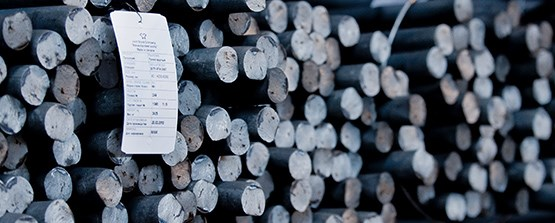sections

# How to Calculate Steel Round Bar Weight

Better to know the formula yourselfSteel Round Bar

While internet offers a lot of calculators for figuring out the weight of steel round bar, it is better to know the formula yourself, as you can’t mindlessly rely on calculators all the time. They do malfunction from time to time. While you may think that the formula for steel round bar calculation is fairly simple (D2 / 162.2) it is not. First of all, you should mind the manufacturer’s chart for calculation the weight. Secondly, there is no exact formula to calculate the weight of steel round bar, no matter how ridiculous it may sound. You may wonder how that’s possible, as there are a lot of websites offering different formulas for calculating the steel round bar weight. But the keyword in this case is “different”. They offer you different formulas. Basically, using one formula you would get one results, and using other formula will provide you with different results. So, regardless of which formula you use, you are most likely to get only approximate results.

So, does it mean that you are left without any formulas for calculating round bar weight? Well, not exactly. There are a few ways to calculate the weight, and we are going to provide you with them. All you have to do is to pick and choose the one you like the best.### 1. The most common formula

The most common formula requires you to know the density for squared inch. According to the formula you can calculate the weight of the round bar this way:

Diameter in inches squared x 2.67 = lbs per foot.

### 2. D2 / 162.2

While this formula can be applied for calculating the round bar weight, it is generally used for calculating the weight of circular rebars. It is the formula for unit weight of steel. To use it, you need to figure out the derivation of D2 / 162.2, which you can see below:

Weight of steel bar per meter = Area of the steel x Density of steel x Bar length

= (3.14 x D2 /4) x 7850 kg/m3 x 1m

= (D2 x 6162.25)/(1000 x 1000)

= D2 X (0.006162)

= D2 X 1/(0.006162)-1

= D2/162.28

3. Experimental method

You can’t avoid experimental methods, when there is no ultimate formula for calculating bar weight. So, to calculate it using the experimental method, you should follow the next steps:

• take a sample length of a steel bar (let it be one feet)
• take the pipe of the same length, but with the larger diameter, so that the bar would fit in it
• fill the pipe with water up to the top
• immerse the bar into the pipe
• then remove the bar from the pipe, and fill the pipe once again with water
• now we can state that the volume of water in the pipe equals the volume of the water that can be placed in the bar

Basically, it is Archimedes theory that we all learned in school.

Steel Round Bar supplier in China, Steel Round Bar supplier in Dubai, Steel Round Bar supplier in Middle East, Steel Round Bar supplier in Singapore, Steel Round Bar supplier in Philippines, Steel Round Bar supplier in Asia, Steel Round Bar supplier in Africa

Read more  What You Need To Know About Steel Frame Construction: The Pros And Cons